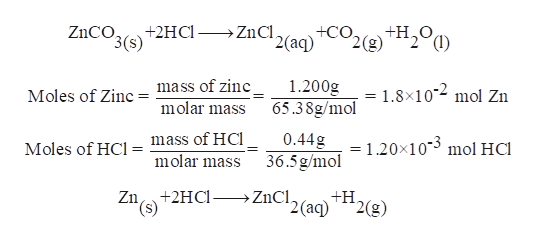# A 1.200 g sample of ZnCO3 (s) dissolved in 40.0 mL of a dilute HCl solution (density = 1.10 g/mL) in a coffee cup calorimeter, producing CO2 (g), liquid water and ZnCl2 (aq).  The temperature of the solution changes from 24.32 oC to 25.49 oC.  The resulting solution has a specific heat of 4.18 J/goC.What would the ∆H for the reaction of one mole of ZnCO3 with HCl as determined by this experiment.

Question
51 views

A 1.200 g sample of ZnCO3 (s) dissolved in 40.0 mL of a dilute HCl solution (density = 1.10 g/mL) in a coffee cup calorimeter, producing CO2 (g), liquid water and ZnCl2 (aq).  The temperature of the solution changes from 24.32 oC to 25.49 oC.  The resulting solution has a specific heat of 4.18 J/goC.

What would the ∆H for the reaction of one mole of ZnCO3 with HCl as determined by this experiment.

check_circle

Step 1

The reactants are zinc carbonate and dilute hydrochlo...help_outlineImage Transcriptionclose+H20 2(g) ZnCO +2HCl ZnCl. 3(s) 2 (аq) Moles of Zinc= mass of zine molar mass 1.200g 65.38g/mol = 1.8x102 mol Zn mass of HCl molar mass 0.44g 36.5g/mol =1.20x103 mol HCl Moles of HCl = Zn+2HC- ZnCl. +H '2(aq) 2(g) *(s) fullscreen

### Want to see the full answer?

See Solution

#### Want to see this answer and more?

Solutions are written by subject experts who are available 24/7. Questions are typically answered within 1 hour.*

See Solution
*Response times may vary by subject and question.
Tagged in

### General Chemistry